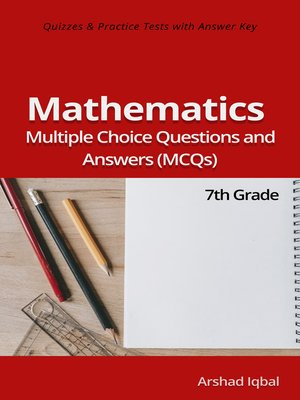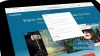### Multiple Choice Questions and Answers (Quiz & Tests with Answer Keys)

ebook

With an OverDrive account, you can save your favorite libraries for at-a-glance information about availability. Find out more about OverDrive accounts.

### Subjects

7th grade math multiple choice questions has 135 MCQs. Grade 7 math quiz questions and answers pdf, MCQs on direct and inverse proportions, surface area and volume, math problems, congruence and similar figures, congruent triangles, quadratic equations, basic mathematics MCQs with answers, set language and notation, algebraic manipulation and formulas, expansion and factorization, algebraic expressions MCQs and quiz are to practice exam prep tests.
7th grade math multiple choice quiz questions and answers pdf, math exam revision and study guide with practice tests for online exam prep and interviews. Math interview questions and answers to ask, to prepare and to study for jobs interviews and career MCQs with answer keys.
Algebraic manipulation and formulas quiz has 22 multiple choice questions. Class 7 math problems quiz has 33 multiple choice questions. Congruence and similarity quiz has 14 multiple choice questions with answers. Direct and inverse proportions quiz has 21 multiple choice questions.
Expansion and factorization of algebraic expressions quiz has 18 multiple choice questions. Set language and notation quiz has 13 multiple choice questions. Volume and surface area quiz has 14 multiple choice questions with answers.
Math interview questions and answers pdf, MCQs on pyramids, Pythagoras theorem, mean median mode, volume of sphere, volume of cones, subsets, surface area of sphere, volume of pyramid, algebraic fractions, direct proportion, direct proportions, inverse proportion, surface area of pyramid, union of sets, intersection of sets, introduction to probability, complement of set, factorization of algebraic expression, graph of equations, introduction to sets, subject of formula, expansion of algebraic expression, quadratic equation in two variables, factorization of quadratic expression, number of elements in set, solving quadratic equation by factorization, algebraic function and equations, cones and surface area, congruent figures and objects, direct proportion and graphs, factorization using algebraic identities, finding unknown in formula, forms of direct proportion, multiplication and division of algebraic fraction, perfect squares and difference, problem solving with algebraic fraction, problem solving with quadratic equation, problem solving with simultaneous equation, similar figures and objects, similarity and scale drawings, simple algebraic fraction, simultaneous linear equation and elimination method, grade 7 math worksheets for competitive exams preparation.

### Publication Details

Publisher:
Imprint:
Smashwords Edition
Publication Date:
2016

### Format